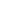Share

# Real Number

Example: "Real numbers are sometimes described as points on an infinite number line."

Real numbers include all positive and negative numbers. They may be integers (e.g. -12), rational numbers (e.g. 9/10), or irrational numbers, (e.g. 1.4142135...). Numbers with decimal points are also called floating point numbers, since the decimal may also be referred to as a floating point. CPUs can process all types of real numbers, though most processors include a separate floating point unit, or FPU, for processing floating point calculations.

So if there are real numbers, do fake numbers exist? Well, considering that fake numbers, by definition, are not real, the answer is no. However, as many computer nerds know, it is still possible for someone to give you a fake phone number.

Published: April 26, 2010

Definition from the PC Glossary
https://pc.net/glossary/realnumber